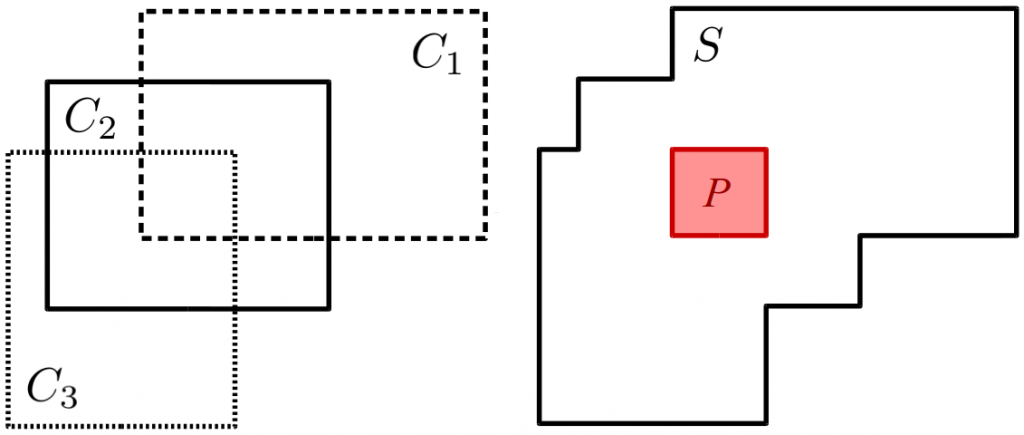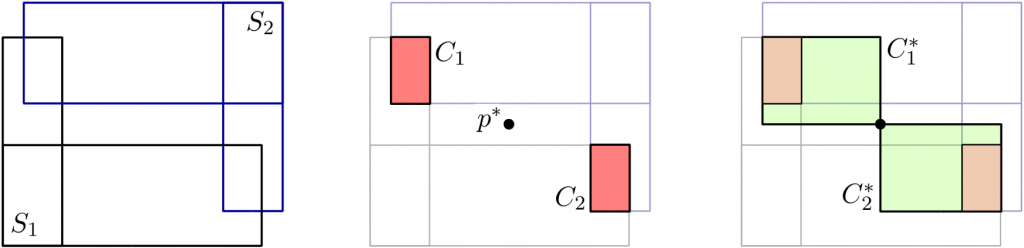# Formalizing Conceptual Spaces With Simple Star-Shaped Sets

In the last two blog posts (see here and here), I have described the problem I address in my paper “A Thorough Formalization of Conceptual Spaces” : Convex regions in a conceptual space are unable to encode correlations between domains. I have furthermore argued that star-shaped regions are a possible solution to this problem.

In this blog post, I would like to illustrate the basic idea of my formalization. I won’t address the underlying math too much (whoever is interested can check out the paper), because my main goal is to give you some intuitive understanding of what’s going on.

The question that was still left open last time is the following one: How exactly can we represent star-shaped regions in a conceptual space?

My formalization is based on so-called “axis-parallel cuboids”. In two dimensions, those are basically rectangles whose sides are parallel to the x- and y-axes. In three dimensions, they have the form of boxes – again with their sides parallel to the x-, y-, and z-axes.

Why did I pick cuboids as a starting point?

Well, there are two reasons: Firstly, we already know that axis-parallel cuboids are convex under the Manhattan metric (see this blog post). Secondly, one can describe them easily: You only need the coordinates of the bottom left point and the coordinates of the top right point to describe a rectangle in two dimensions. The nice thing is that this also holds true in higher-dimensional spaces. For instance, in 3D, we can describe a box by the coordinates of two opposing corners. So even if our conceptual space has twenty or thirty dimensions, we still need to store only two points. This is quite important if we want to write a computer program based on this formalization.

Okay, so we now have these cuboids. But as argued above, they are convex with respect to the Manhattan metric, not star-shaped. How can we get to star-shaped sets from here? Well, the answer is fortunately quite simple: Whenever we have two or more cuboids that overlap, then we can unify them and the result will be star-shaped with respect to the region of overlap. The following figure illustrates this:Figure 1: Three cuboids that form a simple star-shaped set.

The left part of Figure 1 shows three cuboids that overlap (i.e., there are some points that belong to all three cuboids). The right part of Figure 1 shows the set S that results from unifying the three cuboids. The region P highlighted within S is just the region where the three cuboids overlap. I hope you can see that no matter which point x in P you pick, and no matter which point z in S you pick, the rectangle between x and z will always stay within S. This is exactly the definition of star-shapedness under the Manhattan distance.

I call sets like these “simple star-shaped sets”. The word “simple” is supposed to emphasize that these sets are based on simple cuboids and that they are only a special kind of star-shaped sets (there are also other kinds of star-shaped sets, for instance the ones sketched in the previous post).

So basically, I propose to represent each concept by one of these simple star-shaped sets. It is relatively straightforward to implement this in a computer program as you only need to store two points for each cuboid. Of course, you also need to make sure that the different cuboids do overlap, but that is also relatively straightforward.

Now that we have a more concrete way of representing concepts, let’s see whether we can perform some operations on them. The intersection of two concepts would be an example of such an operation. You can think of this as a logical “AND”: If you want to know which objects are both green and blue, you have to find the set of points that belongs to both the “green” region and the “blue” region. This is equivalent to the intersection of these two regions.Figure 2: The different steps of intersecting two simple star-shaped sets.

Figure 2 illustrates how the intersection of two simple star-shaped sets works. In the left part of Figure 2, we see two simple star-shaped sets, S1 and S2. The middle part of Figure 2 shows what happens when we intersect them – we get two cuboids, namely C1 and C2. However, those two cuboids do not overlap. This is a bit problematic, because we would like to get a concept as a result of this intersection – that is, a simple star-shaped set. So what do we do?

Well, a simple workaround looks as follows: We pick a point p* between the two cuboids (also shown in the middle part of Figure 2). We then extend each cuboid, such that it contains this point p* (shown in the right part of Figure 2). And voilà – we have a simple star-shaped set again, because now there exists a point (namely p*) that belongs to both extended cuboids C*1 and C*2.

One can also define the union of two simple star-shaped sets in a similar way: If the cuboids do not overlap, pick a midpoint p* and extend them such that they meet in p*.

I think this already illustrates the basic idea of my formalization reasonably well. There’s one little detail left, and I’ll cover it in my next blog post. It is the problem of imprecise concept boundaries. What is this about and how do I deal with it? Stay tuned…

###### References

 Bechberger, Lucas and Kühnberger, Kai-Uwe. “A Thorough Formalization of Conceptual Spaces” 40th German Conference on Artificial Intelligence, 2017.